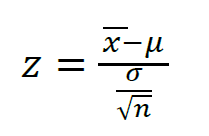# Tag Archives: one sample z test# What is a One Sample z Test?

There are actually several different situations in which a researcher can use hypothesis testing. The first instance we will look at is the one sample z test. The one sample z test has the following assumptions that need to be met before employing it.

• Sample size > 30
• Subjects are randomly selected
• Population is normally distributed
• Cases within the sample are independent
• One sample was taken

If your data collection meets the above assumptions one sample z test may be appropriate.

With the one sample z test, you are comparing your results to a known expectedvalue. For example, if someone states that the average salaries for teachers are \$63,000.00 you can assess this by collecting data from teachers to compare it to this known value. You collect some data and you find that the average salary for 35 teachers was \$65,7000.00. The questions you have is who is right? Do teachers really make on average \$63,000.00 like the report or do they make \$65,700.00 as my data says? Before going further let us establish are hypotheses for this example.

• Null hypothesis: the average salaries for my sample of teacher salaries will be the same as the average salary’s of the reported value of \$63,000.00
1. The mathematical shorthand for this is H0: μ = 63,000.00
• Alternative hypothesis: the average salaries for my sample of teacher salaries will be different (greater or lesser) than the average salary’s of the reported value of \$63,000.00
1. The mathematical shorthand for this is H1: μ ≠ 63,000.00

Keep in mind that this is a two-tail level of significance. This is because our final value has the option of being either greater or lesser than \$63,000.00. Two-tail means two options, greater or lesser than the expected value while one-tail means only one option either we expect greater or we expected lesser but not both. This is why we will have two z critical values to think about in the near future.

We also need two more pieces of information before we put our numbers into the equation. The two items we need to know are the standard deviation of the sample and the level of statistical significance. For the sample data, we collected we will say the standard deviation is \$5,250.00 and the level of statistical significance is α = 0.01. When we convert this alpha value to the z critical value we get 2.32 and -2.32 because we are using a two-tail or two option approach. Do not get distracted by the z critical value it is the same as the alpha value but translated for the numbers set to the normal distribution. It is similar to switching from one language to another, same meaning but different language.

If our final value is greater than 2.32 or less than -2.32 we will reject the null hypothesis that average teacher salaries are \$63,000.00. Now we can take a look at the equation

z critical value = sample data – expected value                                                                                           Sample standard deviation / square root of the                                                    number of those in the sample population

In simple English

z critical value  = 65,700 – 63,000                                                                                                                         5,250 / square root of 35

Z critical value = 3.04

Our answer is 3.04, which is greater than +2.32. This indicates that we can reject the null hypothesis that the average salary teachers are \$63,000.00 as our data indicate that there is evidence that teachers make more on average.

We don’t want to get too excited here. We found evidence that teachers make more but further testing would be needed to validate these claims. As more data confirms our findings we can confidently state that teachers make more.

I would like to thank andydevil12 for the question and suggestion. If there are any other questions please send them to me as they help me to understand research and statistics much better as well.